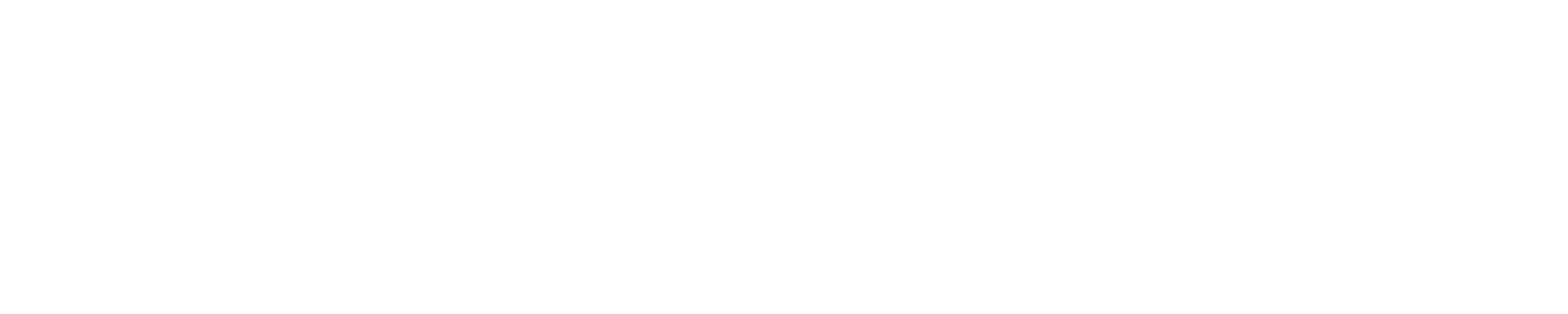# Template loop detected in template sensor performing cumulative sum

Tags: #<Tag:0x00007fc3f840cf68>

Hi i hope i’m having a template loop detected warning using template sensor in configuration.yaml to perform cumulative sum. I probably can solve it using trigger, but trying to keep it simple.

here’s part of the warning.

WARNING (MainThread) [homeassistant.components.template.template_entity] Template loop detected while processing event: <Event state_changed[L]: entity_id=sensor.inverter_121810025597_sum, old_state=<state sensor.inverter_121810025597_sum=1976; unit_of_measurement=W, friendly_name=inverter_121810025597_sum @ 2021-10-10T13:10:14.844231-05:00>, new_state=<state sensor.inverter_121810025597_sum=2060; unit_of_measurement=W, friendly_name=inverter_121810025597_sum @ 2021-10-10T13:10:17.016148-05:00>>, skipping template render for Template[{% set hour = now().strftime(’%H’)|int(0) %} {% set prev_totprod1= states(‘sensor.inverter_121810025597_sum’)|int(0) %} {% if (hour>=0 and hour<=6) %}

here’s my template sensor

``````      inverter_121810025597_sum:
friendly_name: 'inverter_121810025597_sum'
value_template: >
{% set hour = now().strftime('%H')|int(0) %}
{% set prev_totprod1= states('sensor.inverter_121810025597_sum')|int(0) %}
{% if (hour>=0 and hour<=6) %}
{% set totprod= 0 %}
{% else %}
{% set totprod1= states('sensor.envoy_inverters_inverter_121810025597') %}
{% if (totprod1==unknown) or (totprod1|int(0)<0) %}
{% set totprod=prev_totprod1 %}
{% else %}
{% set totprod=totprod1|int(0)+prev_totprod1 %}
{% endif %}
{% endif %}
{{ totprod|int(0) }}
``````

If there is a simple fix to prevent loop detection, it’ll be appreciated
otherwise i’ll have to complicate it and add a trigger to perform the sum
and still have a template sensor so i can use it to display the sum in a history graph.

Thanks

in case others are running into this issue, I was inspired with a solution from the following

my goal was to eliminate the log Warnings, that make it hard to see serious errors in the home-assistant.log file. I also added logic to replace negative values with 0, not related to my original post. My new template sensor is complicated, but I had to rewrite it to make the log warnings go away.

here my new template sensor

``````      inverter_121810025597_sum:
friendly_name: 'inverter_121810025597_sum'
value_template: >
{% set hour = now().hour|int(0) %}
{% if (hour>=0 and hour<=6) %}
{{ 0|int }}
{% else %}
{% set prod = [ ([0,states.sensor.inverter_121810025597_sum.state|default(0)|int]|sort), ([0,states.sensor.envoy_inverters_inverter_121810025597.state|default(0)|int]|sort) ] | list %}
{{ prod |map('int')| sum | int }}
{% endif %}
unit_of_measurement: 'W'
``````
``````      inverter_121810025597_sum:
friendly_name: 'inverter_121810025597_sum'
value_template: >
{{ 0 if 0 <= now().hour <= 6 else ([0, states('sensor.inverter_121810025597_sum') | int(0)] | max * 2) | int(0) }}
unit_of_measurement: 'W'``````